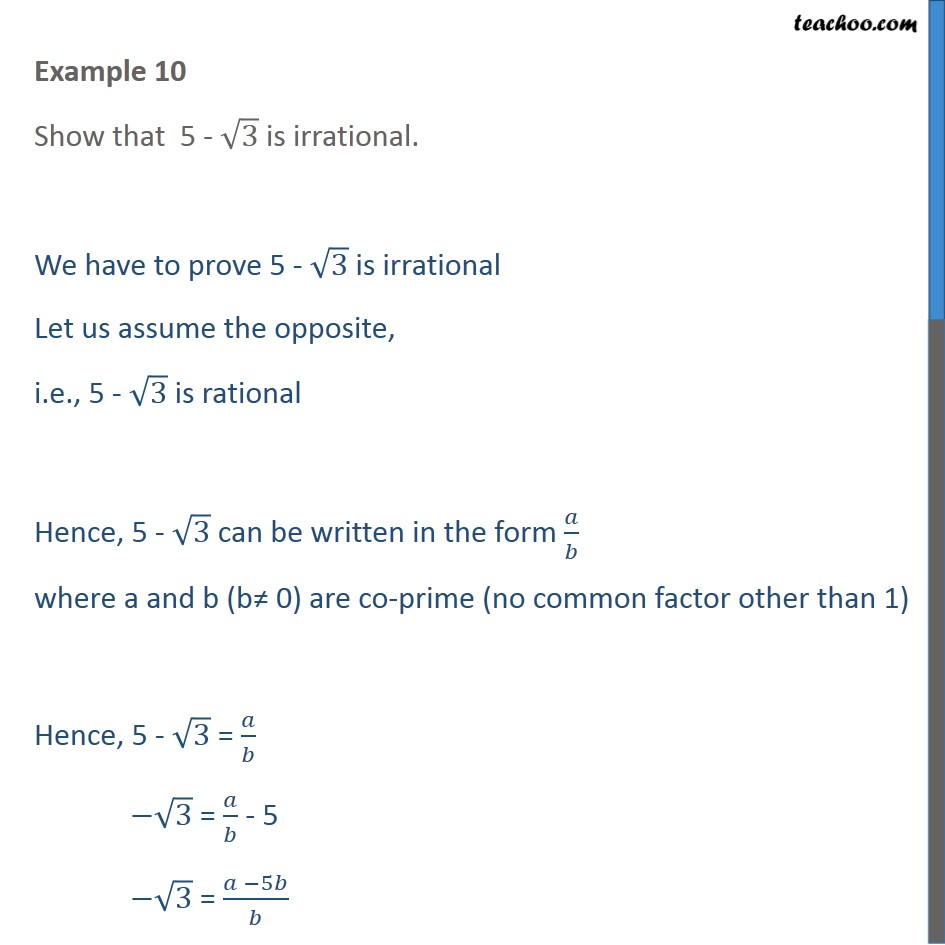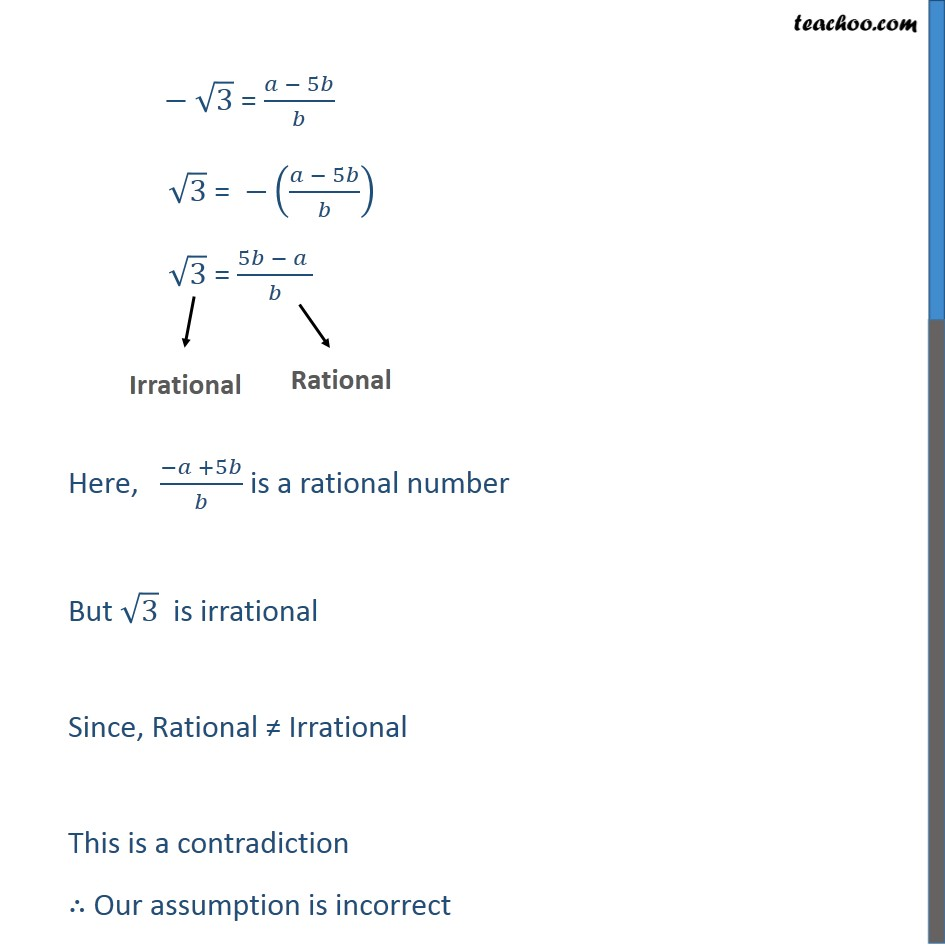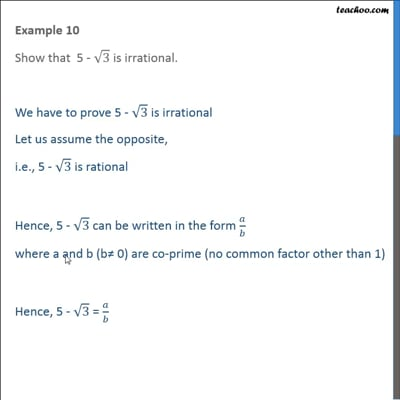Examples

Chapter 1 Class 10 Real Numbers (Term 1)
Serial order wiseThis video is only available for Teachoo black users

### Transcript

Example 10 Show that 5 - 3 is irrational. We have to prove 5 - 3 is irrational Let us assume the opposite, i.e., 5 - 3 is rational Hence, 5 - 3 can be written in the form / where a and b (b 0) are co-prime (no common factor other than 1) Hence, 5 - 3 = / 3 = / - 5 3 = ( 5 )/ 3 = ( 5 )/ 3 = (( 5 )/ ) 3 = (5 )/ Here, ( +5 )/ is a rational number But 3 is irrational Since, Rational Irrational This is a contradiction Our assumption is incorrect Hence, 5 - 3 is irrational Hence proved.